# Liquidity Ratios What are liquidity ratios Ratios derived

• Slides: 19Liquidity RatiosWhat are liquidity ratios? Ratios derived from the balance sheet that measure how easily a firm can pay its debtsThe balance sheet • These ratios are derived from the balance sheet • The balance sheet of a business provides a snapshot of the assets and liabilities at a particular point in time • For these ratios we focus on working capital items: current assets and current liabilities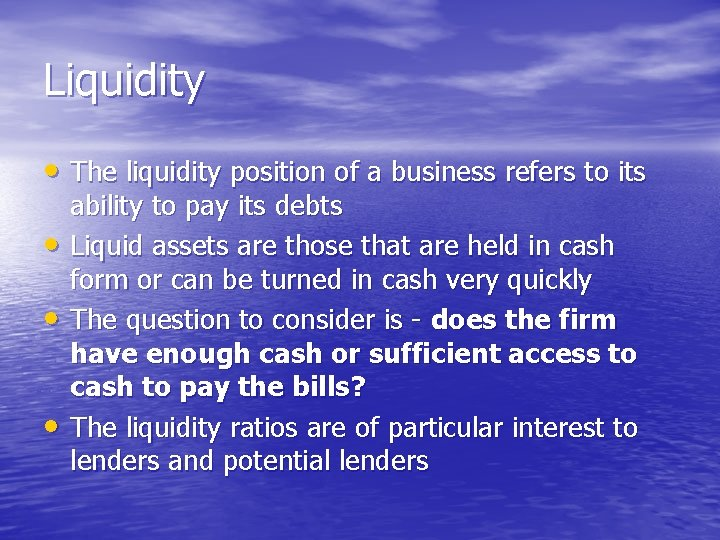Liquidity • The liquidity position of a business refers to its • • • ability to pay its debts Liquid assets are those that are held in cash form or can be turned in cash very quickly The question to consider is - does the firm have enough cash or sufficient access to cash to pay the bills? The liquidity ratios are of particular interest to lenders and potential lendersLiquid assets • Current assets are defined as receivable within 12 months. As a result they are seen as liquid assets • Current or liquid assets are those assets that are held in a cash form and those assets that can be turned into cash very quickly • Debtors (money promised to the business) can be quickly turned into cash • Stock can be sold off and turned into cash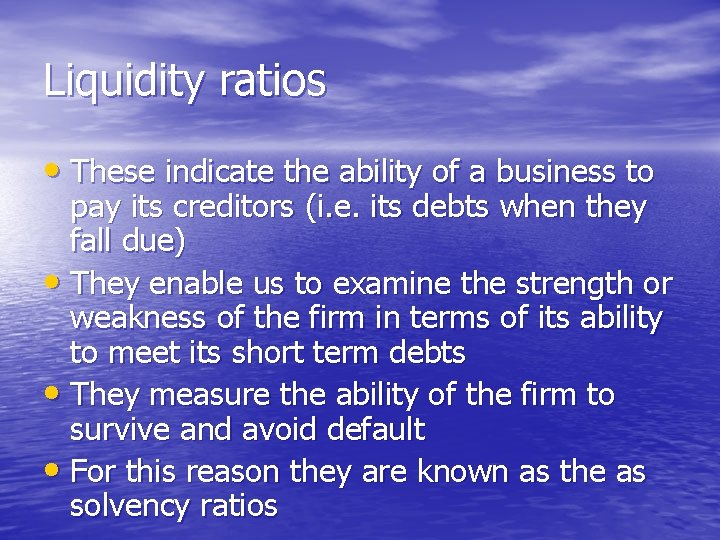Liquidity ratios • These indicate the ability of a business to pay its creditors (i. e. its debts when they fall due) • They enable us to examine the strength or weakness of the firm in terms of its ability to meet its short term debts • They measure the ability of the firm to survive and avoid default • For this reason they are known as the as solvency ratiosTwo key ratios • There are two key ratios that can be calculated to provide a guide to the liquidity position of a business: • The current ratio (or current acid ratio) current assets /current liabilities • The acid test or quick ratio current assets less stock /current liabilities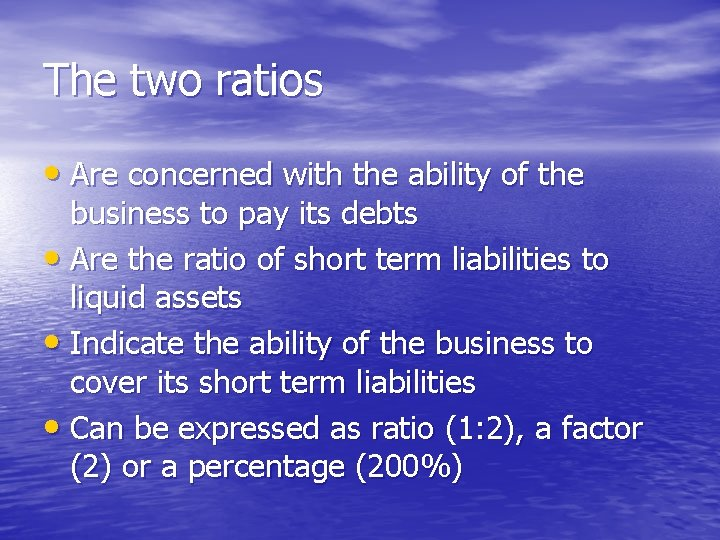The two ratios • Are concerned with the ability of the business to pay its debts • Are the ratio of short term liabilities to liquid assets • Indicate the ability of the business to cover its short term liabilities • Can be expressed as ratio (1: 2), a factor (2) or a percentage (200%)Interpreting the ratios • A business needs to have enough cash or cash to come to be able to pay its debts • A current ratio in excess of one should be expected (although firms in some industries manage with less) • An acid test ratio of around one is also desirable • It will mean that the firm will have little difficulty in meeting its debtsCurrent ratio • This ratios is a test of liquidity which determines • • • whether short term assets cover short term liabilities Calculation: current assets divided by current liabilities Or stock + debtors + cash divided by current liabilities It shows how many times current liabilities are covered by current assets A figure of one means that CA exactly equal CL. A figure of 1. 5 means that CA assets cover CL one and a half times overInterpreting the Current ratio • The textbook ideal is somewhere between 1. 5 and 2 but this is only a guide • If it is lower, it means there is a danger of insufficient cash to pay off debts as they fall due - especially if a high proportion of current assets consists of stocks • If it is higher, it suggests that too much money is tied up in stock or left idle as cash or debtorsAcid test (or quick) ratio • This is a measure of extreme short term liquidity • It is similar to the current ratio but it excludes stock • This makes it a tougher test of liquidity • Calculation: – current assets less stock divided by current liabilities OR – cash plus debtors divided by current liabilitiesWhy exclude stock? • Stock takes longer to turn into cash • Stock is the least liquid of current assets • The firm will be reluctant to sell off stock in a rush - to do so will mean reducing price and therefore reducing profits • The acid test is a measure of whether the business can meet its short-term debts without selling off any stock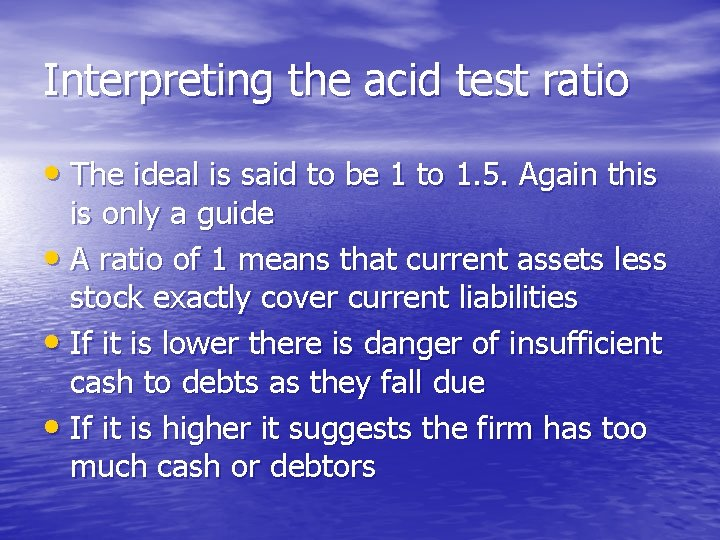Interpreting the acid test ratio • The ideal is said to be 1 to 1. 5. Again this is only a guide • A ratio of 1 means that current assets less stock exactly cover current liabilities • If it is lower there is danger of insufficient cash to debts as they fall due • If it is higher it suggests the firm has too much cash or debtorsBut it is all relative • Some businesses find it hard to turn stock and • • • debtors into cash and so need a high ratio Some businesses such as supermarkets turn stock into cash very rapidly - so they can happily exist with a current ratio of less than one and even as low as 0. 3 Large firms are powerful enough to delay payment to suppliers and therefore can happily operate with low liquidity ratios The acid test is a better test of liquidity for businesses with a low stock turnoverLimitations of liquidity ratios • Like all ratios they should be used with care • The liquidity ratios are derived from the balance sheet • • • which in turn is a snapshot at a moment in time. Balance sheet values at a particular moment in time may not be typical Ratios can be subject to window dressing or manipulation Ratios concern the past and not the future Ratios do not tells what is going right or wrong-they merely invite further questions Liquidity ratios will vary with seasonal fluctuations in sales What is satisfactory will depend on the nature of the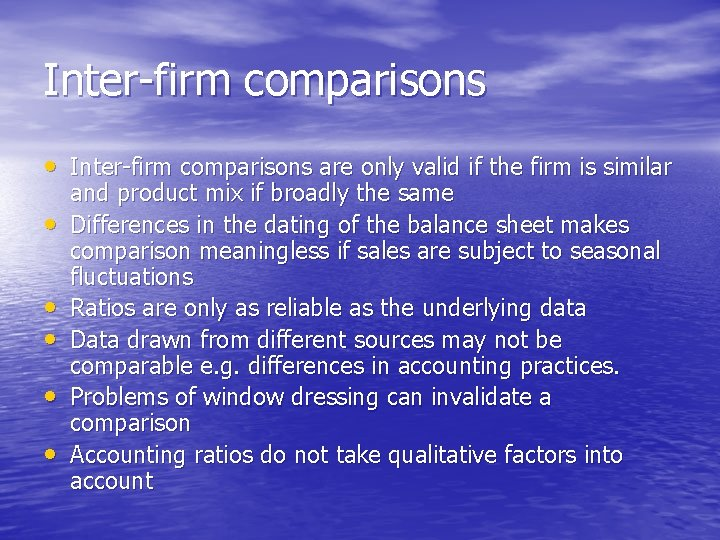Inter-firm comparisons • Inter-firm comparisons are only valid if the firm is similar • • • and product mix if broadly the same Differences in the dating of the balance sheet makes comparison meaningless if sales are subject to seasonal fluctuations Ratios are only as reliable as the underlying data Data drawn from different sources may not be comparable e. g. differences in accounting practices. Problems of window dressing can invalidate a comparison Accounting ratios do not take qualitative factors into account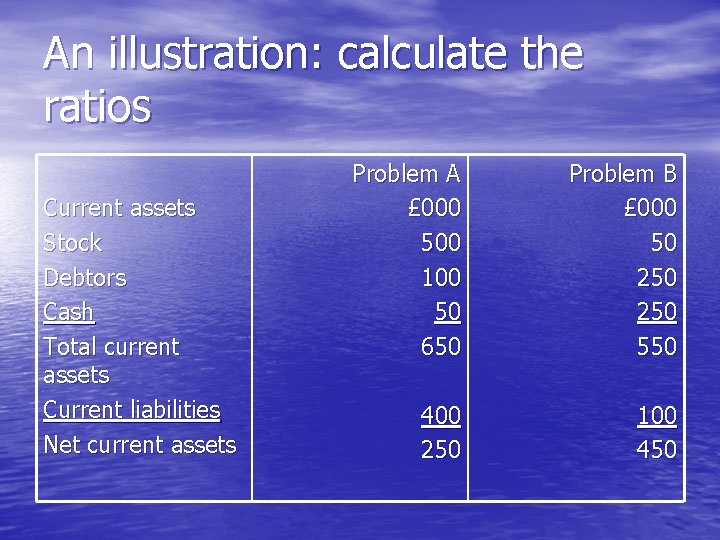An illustration: calculate the ratios Current assets Stock Debtors Cash Total current assets Current liabilities Net current assets Problem A £ 000 500 100 50 650 Problem B £ 000 50 250 550 400 250 100 450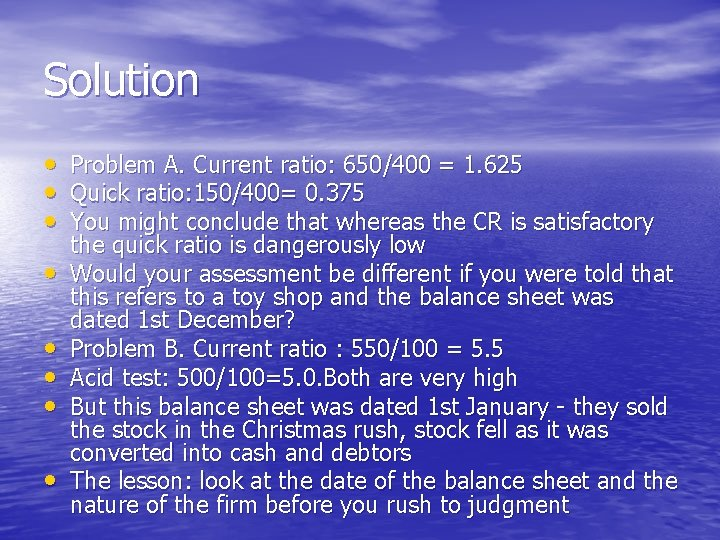Solution • Problem A. Current ratio: 650/400 = 1. 625 • Quick ratio: 150/400= 0. 375 • You might conclude that whereas the CR is satisfactory • • • the quick ratio is dangerously low Would your assessment be different if you were told that this refers to a toy shop and the balance sheet was dated 1 st December? Problem B. Current ratio : 550/100 = 5. 5 Acid test: 500/100=5. 0. Both are very high But this balance sheet was dated 1 st January - they sold the stock in the Christmas rush, stock fell as it was converted into cash and debtors The lesson: look at the date of the balance sheet and the nature of the firm before you rush to judgment